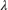# Implement Hardware-Efficient Complex Burst Matrix Solve Using QR Decomposition with Tikhonov Regularization

This example shows how to use the Complex Burst Matrix Solve Using QR Decomposition block to solve the regularized least-squares matrix equationwhere A is an m-by-n matrix with m >= n, B is m-by-p, X is n-by-p,eye(n),zeros(n,p), andis a regularization parameter.

The least-squares solution isbut is computed without squares or inverses.

### Define Matrix Dimensions

Specify the number of rows in matrices A and B, the number of columns in matrix A, and the number of columns in matrix B.

m = 100; % Number of rows in matrices A and B n = 10; % Number of columns in matrix A p = 1; % Number of columns in matrix B 

### Define Tikhonov Regularization Parameter

Small, positive values of the regularization parameter can improve the conditioning of the problem and reduce the variance of the estimates. While biased, the reduced variance of the estimate often results in a smaller mean squared error when compared to least-squares estimates.

regularizationParameter = 0.01; 

### Generate Random Least-Squares Matrices

For this example, use the helper function complexRandomLeastSquaresMatrices to generate random matrices A and B for the least-squares problem AX=B. The matrices are generated such that the real and imaginary parts of the elements of A and B are between -1 and +1, and A has rank r.

rng('default') r = 3; % Rank of A [A,B] = fixed.example.complexRandomLeastSquaresMatrices(m,n,p,r); 

### Select Fixed-Point Data Types

Use the helper function complexQRMatrixSolveFixedpointTypes to select fixed-point data types for input matrices A and B, and output X such that there is a low probability of overflow during the computation.

The real and imaginary parts of the elements of A and B are between -1 and 1, so the maximum possible absolute value of any element is sqrt(2).

max_abs_A = sqrt(2); % Upper bound on max(abs(A(:)) max_abs_B = sqrt(2); % Upper bound on max(abs(B(:)) precisionBits = 32; % Number of bits of precision T = fixed.complexQRMatrixSolveFixedpointTypes(m,n,max_abs_A,max_abs_B,... precisionBits,[],[],regularizationParameter); A = cast(A,'like',T.A); B = cast(B,'like',T.B); OutputType = fixed.extractNumericType(T.X); 

### Open the Model

model = 'ComplexBurstQRMatrixSolveModel'; open_system(model);The Data Handler subsystem in this model takes complex matrices A and B as inputs. The ready port triggers the Data Handler. After sending a true validIn signal, there may be some delay before ready is set to false. When the Data Handler detects the leading edge of the ready signal, the block sets validIn to true and sends the next row of A and B. This protocol allows data to be sent whenever a leading edge of the ready signal is detected, ensuring that all data is processed.

### Set Variables in the Model Workspace

Use the helper function setModelWorkspace to add the variables defined above to the model workspace. These variables correspond to the block parameters for the Complex Burst Matrix Solve Using QR Decomposition block.

numSamples = 1; % Number of sample matrices fixed.example.setModelWorkspace(model,'A',A,'B',B,'m',m,'n',n,'p',p,... 'regularizationParameter',regularizationParameter,... 'numSamples',numSamples,'OutputType',OutputType); 

### Simulate the Model

out = sim(model); 

### Construct the Solution from the Output Data

The Complex Burst Matrix Solve Using QR Decomposition block outputs data one row at a time. When a result row is output, the block sets validOut to true. The rows of X are output in the order they are computed, last row first, so you must reconstruct the data to interpret the results. To reconstruct the matrix X from the output data, use the helper function matrixSolveModelOutputToArray.

X = fixed.example.matrixSolveModelOutputToArray(out.X,n,p,numSamples); 

### Verify the Accuracy of the Output

Verify that the relative error between the fixed-point output and builtin MATLAB in double-precision floating-point is small.A_lambda = double([regularizationParameter*eye(n);A]); B_0 = [zeros(n,p);double(B)]; X_double = A_lambda\B_0; relativeError = norm(X_double - double(X))/norm(X_double) 
relativeError = 2.7722e-05 

Suppress mlint warnings in this file.

%#ok<*NOPTS> %#ok<*NASGU> %#ok<*ASGLU>